Rigid Body Dynamics Library
Kinematics

## Data Structures

struct  InverseKinematicsConstraintSet

## Functions

RBDL_DLLAPI void UpdateKinematics (Model &model, const Math::VectorNd &Q, const Math::VectorNd &QDot, const Math::VectorNd &QDDot)
Updates and computes velocities and accelerations of the bodies. More...

RBDL_DLLAPI void UpdateKinematicsCustom (Model &model, const Math::VectorNd *Q, const Math::VectorNd *QDot, const Math::VectorNd *QDDot)
Selectively updates model internal states of body positions, velocities and/or accelerations. More...

RBDL_DLLAPI Vector3d CalcBodyToBaseCoordinates (Model &model, const Math::VectorNd &Q, unsigned int body_id, const Math::Vector3d &body_point_position, bool update_kinematics=true)
Returns the base coordinates of a point given in body coordinates. More...

RBDL_DLLAPI Vector3d CalcBaseToBodyCoordinates (Model &model, const Math::VectorNd &Q, unsigned int body_id, const Math::Vector3d &base_point_position, bool update_kinematics=true)
Returns the body coordinates of a point given in base coordinates. More...

RBDL_DLLAPI Matrix3d CalcBodyWorldOrientation (Model &model, const Math::VectorNd &Q, const unsigned int body_id, bool update_kinematics=true)
Returns the orientation of a given body as 3x3 matrix. More...

RBDL_DLLAPI void CalcPointJacobian (Model &model, const Math::VectorNd &Q, unsigned int body_id, const Math::Vector3d &point_position, Math::MatrixNd &G, bool update_kinematics=true)
Computes the point jacobian for a point on a body. More...

RBDL_DLLAPI void CalcPointJacobian6D (Model &model, const Math::VectorNd &Q, unsigned int body_id, const Math::Vector3d &point_position, Math::MatrixNd &G, bool update_kinematics=true)
Computes a 6-D Jacobian for a point on a body. More...

RBDL_DLLAPI void CalcBodySpatialJacobian (Model &model, const Math::VectorNd &Q, unsigned int body_id, Math::MatrixNd &G, bool update_kinematics=true)
Computes the spatial jacobian for a body. More...

RBDL_DLLAPI Vector3d CalcPointVelocity (Model &model, const Math::VectorNd &Q, const Math::VectorNd &QDot, unsigned int body_id, const Math::Vector3d &point_position, bool update_kinematics=true)
Computes the velocity of a point on a body. More...

RBDL_DLLAPI Math::SpatialVector CalcPointVelocity6D (Model &model, const Math::VectorNd &Q, const Math::VectorNd &QDot, unsigned int body_id, const Math::Vector3d &point_position, bool update_kinematics=true)
Computes angular and linear velocity of a point that is fixed on a body. More...

RBDL_DLLAPI Vector3d CalcPointAcceleration (Model &model, const Math::VectorNd &Q, const Math::VectorNd &QDot, const Math::VectorNd &QDDot, unsigned int body_id, const Math::Vector3d &point_position, bool update_kinematics=true)
Computes the linear acceleration of a point on a body. More...

RBDL_DLLAPI SpatialVector CalcPointAcceleration6D (Model &model, const Math::VectorNd &Q, const Math::VectorNd &QDot, const Math::VectorNd &QDDot, unsigned int body_id, const Math::Vector3d &point_position, bool update_kinematics=true)
Computes linear and angular acceleration of a point on a body. More...

RBDL_DLLAPI bool InverseKinematics (Model &model, const Math::VectorNd &Qinit, const std::vector< unsigned int > &body_id, const std::vector< Math::Vector3d > &body_point, const std::vector< Math::Vector3d > &target_pos, Math::VectorNd &Qres, double step_tol=1.0e-12, double lambda=0.01, unsigned int max_iter=55)
Computes the inverse kinematics iteratively using a damped Levenberg-Marquardt method (also known as Damped Least Squares method) More...

RBDL_DLLAPI Vector3d CalcAngularVelocityfromMatrix (const Matrix3d &RotMat)

RBDL_DLLAPI bool InverseKinematics (Model &model, const Math::VectorNd &Qinit, InverseKinematicsConstraintSet &CS, Math::VectorNd &Qres)

## Detailed Description

Note
Please note that in the Rigid Body Dynamics Library all angles are specified in radians.

## ◆ CalcAngularVelocityfromMatrix()

 RBDL_DLLAPI Math::Vector3d CalcAngularVelocityfromMatrix ( const Matrix3d & RotMat )

Definition at line 699 of file Kinematics.cc.

References std::atan2(), and RigidBodyDynamics::Math::Vector3dZero.

## ◆ CalcBaseToBodyCoordinates()

 RBDL_DLLAPI Math::Vector3d CalcBaseToBodyCoordinates ( Model & model, const Math::VectorNd & Q, unsigned int body_id, const Math::Vector3d & base_point_position, bool update_kinematics = true )

Returns the body coordinates of a point given in base coordinates.

Parameters
 model the rigid body model Q the curent genereralized positions body_id id of the body for which the point coordinates are expressed base_point_position coordinates of the point in base coordinates update_kinematics whether UpdateKinematics() should be called or not (default: true).
Returns
a 3-D vector with coordinates of the point in body coordinates

Definition at line 190 of file Kinematics.cc.

References RigidBodyDynamics::UpdateKinematicsCustom().

## ◆ CalcBodySpatialJacobian()

 RBDL_DLLAPI void CalcBodySpatialJacobian ( Model & model, const Math::VectorNd & Q, unsigned int body_id, Math::MatrixNd & G, bool update_kinematics = true )

Computes the spatial jacobian for a body.

The spatial velocity of a body at the origin of coordinate system of body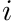can be expressed as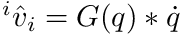. The matrix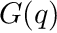is called the spatial body jacobian of the body and can be computed using this function.

Parameters
 model rigid body model Q state vector of the internal joints body_id the id of the body G a matrix of size 6 x #qdot_size where the result will be stored in update_kinematics whether UpdateKinematics() should be called or not (default: true)

The result will be returned via the G argument and represents the body Jacobian expressed at the origin of the body.

Note
This function only evaluates the entries of G that are non-zero. One Before calling this function one has to ensure that all other values have been set to zero, e.g. by calling G.setZero().

Definition at line 370 of file Kinematics.cc.

## ◆ CalcBodyToBaseCoordinates()

 RBDL_DLLAPI Math::Vector3d CalcBodyToBaseCoordinates ( Model & model, const Math::VectorNd & Q, unsigned int body_id, const Math::Vector3d & body_point_position, bool update_kinematics = true )

Returns the base coordinates of a point given in body coordinates.

Parameters
 model the rigid body model Q the curent genereralized positions body_id id of the body for which the point coordinates are expressed body_point_position coordinates of the point in body coordinates update_kinematics whether UpdateKinematics() should be called or not (default: true)
Returns
a 3-D vector with coordinates of the point in base coordinates

Definition at line 157 of file Kinematics.cc.

References RigidBodyDynamics::UpdateKinematicsCustom().

## ◆ CalcBodyWorldOrientation()

 RBDL_DLLAPI Math::Matrix3d CalcBodyWorldOrientation ( Model & model, const Math::VectorNd & Q, const unsigned int body_id, bool update_kinematics = true )

Returns the orientation of a given body as 3x3 matrix.

Parameters
 model the rigid body model Q the curent genereralized positions body_id id of the body for which the point coordinates are expressed update_kinematics whether UpdateKinematics() should be called or not (default: true).
Returns
An orthonormal 3x3 matrix that rotates vectors from base coordinates to body coordinates.

Definition at line 222 of file Kinematics.cc.

References RigidBodyDynamics::UpdateKinematicsCustom().

## ◆ CalcPointAcceleration()

 RBDL_DLLAPI Math::Vector3d CalcPointAcceleration ( Model & model, const Math::VectorNd & Q, const Math::VectorNd & QDot, const Math::VectorNd & QDDot, unsigned int body_id, const Math::Vector3d & point_position, bool update_kinematics = true )

Computes the linear acceleration of a point on a body.

Parameters
 model rigid body model Q state vector of the internal joints QDot velocity vector of the internal joints QDDot velocity vector of the internal joints body_id the id of the body point_position the position of the point in body-local data update_kinematics whether UpdateKinematics() should be called or not (default: true)
Returns
The cartesian acceleration of the point in global frame (output)

The kinematic state of the model has to be updated before valid values can be obtained. This can either be done by calling UpdateKinematics() or setting the last parameter update_kinematics to true (default).

Warning
If this function is called after ForwardDynamics() without an update of the kinematic state one has to add the gravity acceleration has to be added to the result.

Definition at line 515 of file Kinematics.cc.

## ◆ CalcPointAcceleration6D()

 RBDL_DLLAPI Math::SpatialVector CalcPointAcceleration6D ( Model & model, const Math::VectorNd & Q, const Math::VectorNd & QDot, const Math::VectorNd & QDDot, unsigned int body_id, const Math::Vector3d & point_position, bool update_kinematics = true )

Computes linear and angular acceleration of a point on a body.

Parameters
 model rigid body model Q state vector of the internal joints QDot velocity vector of the internal joints QDDot velocity vector of the internal joints body_id the id of the body point_position the position of the point in body-local data update_kinematics whether UpdateKinematics() should be called or not (default: true)
Returns
A 6-D vector where the first three elements are the angular acceleration and the last three elements the linear accelerations of the point.

The kinematic state of the model has to be updated before valid values can be obtained. This can either be done by calling UpdateKinematics() or setting the last parameter update_kinematics to true (default).

Warning
If this function is called after ForwardDynamics() without an update of the kinematic state one has to add the gravity acceleration has to be added to the result.

Definition at line 562 of file Kinematics.cc.

## ◆ CalcPointJacobian()

 RBDL_DLLAPI void CalcPointJacobian ( Model & model, const Math::VectorNd & Q, unsigned int body_id, const Math::Vector3d & point_position, Math::MatrixNd & G, bool update_kinematics = true )

Computes the point jacobian for a point on a body.

If a position of a point is computed by a function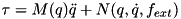for which its time derivative is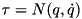then this function computes the jacobian matrix.

Parameters
 model rigid body model Q state vector of the internal joints body_id the id of the body point_position the position of the point in body-local data G a matrix of dimensions 3 x #qdot_size where the result will be stored in update_kinematics whether UpdateKinematics() should be called or not (default: true)

The result will be returned via the G argument.

Note
This function only evaluates the entries of G that are non-zero. One Before calling this function one has to ensure that all other values have been set to zero, e.g. by calling G.setZero().

Definition at line 244 of file Kinematics.cc.

## ◆ CalcPointJacobian6D()

 RBDL_DLLAPI void CalcPointJacobian6D ( Model & model, const Math::VectorNd & Q, unsigned int body_id, const Math::Vector3d & point_position, Math::MatrixNd & G, bool update_kinematics = true )

Computes a 6-D Jacobian for a point on a body.

Computes the 6-D Jacobianthat when multiplied with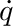gives a 6-D vector that has the angular velocity as the first three entries and the linear velocity as the last three entries.

Parameters
 model rigid body model Q state vector of the internal joints body_id the id of the body point_position the position of the point in body-local data G a matrix of dimensions 6 x #qdot_size where the result will be stored in update_kinematics whether UpdateKinematics() should be called or not (default: true)

The result will be returned via the G argument.

Note
This function only evaluates the entries of G that are non-zero. One Before calling this function one has to ensure that all other values have been set to zero, e.g. by calling G.setZero().

Definition at line 308 of file Kinematics.cc.

## ◆ CalcPointVelocity()

 RBDL_DLLAPI Math::Vector3d CalcPointVelocity ( Model & model, const Math::VectorNd & Q, const Math::VectorNd & QDot, unsigned int body_id, const Math::Vector3d & point_position, bool update_kinematics = true )

Computes the velocity of a point on a body.

Parameters
 model rigid body model Q state vector of the internal joints QDot velocity vector of the internal joints body_id the id of the body point_position the position of the point in body-local data update_kinematics whether UpdateKinematics() should be called or not (default: true)
Returns
The cartesian velocity of the point in global frame (output)

Definition at line 434 of file Kinematics.cc.

## ◆ CalcPointVelocity6D()

 RBDL_DLLAPI Math::SpatialVector CalcPointVelocity6D ( Model & model, const Math::VectorNd & Q, const Math::VectorNd & QDot, unsigned int body_id, const Math::Vector3d & point_position, bool update_kinematics = true )

Computes angular and linear velocity of a point that is fixed on a body.

Parameters
 model rigid body model Q state vector of the internal joints QDot velocity vector of the internal joints body_id the id of the body point_position the position of the point in body-local data update_kinematics whether UpdateKinematics() should be called or not (default: true)
Returns
The a 6-D vector for which the first three elements are the angular velocity and the last three elements the linear velocity in the global reference system.

Definition at line 478 of file Kinematics.cc.

## ◆ InverseKinematics() [1/2]

 RBDL_DLLAPI bool InverseKinematics ( Model & model, const Math::VectorNd & Qinit, const std::vector< unsigned int > & body_id, const std::vector< Math::Vector3d > & body_point, const std::vector< Math::Vector3d > & target_pos, Math::VectorNd & Qres, double step_tol = 1.0e-12, double lambda = 0.01, unsigned int max_iter = 55 )

Computes the inverse kinematics iteratively using a damped Levenberg-Marquardt method (also known as Damped Least Squares method)

Parameters
 model rigid body model Qinit initial guess for the state body_id a vector of all bodies for which we we have kinematic target positions body_point a vector of points in body local coordinates that are to be matched to target positions target_pos a vector of target positions Qres output of the computed inverse kinematics step_tol tolerance used for convergence detection lambda damping factor for the least squares function max_iter maximum number of steps that should be performed
Returns
true on success, false otherwise

This function repeatedly computes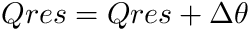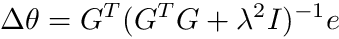where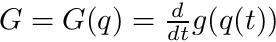and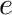is the correction of the body points so that they coincide with the target positions. The function returns true when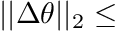step_tol or if the error between body points and target gets smaller than step_tol. Otherwise it returns false.

The parameteris the damping factor that has to be chosen carefully. In case of unreachable positions higher values (e.g 0.9) can be helpful. Otherwise values of 0.0001, 0.001, 0.01, 0.1 might yield good results. See the literature for best practices.

Warning
The actual accuracy might be rather low (~1.0e-2)! Use this function with a grain of suspicion.

Definition at line 605 of file Kinematics.cc.

## ◆ InverseKinematics() [2/2]

 RBDL_DLLAPI bool InverseKinematics ( Model & model, const Math::VectorNd & Qinit, InverseKinematicsConstraintSet & CS, Math::VectorNd & Qres )

## ◆ UpdateKinematics()

 RBDL_DLLAPI void UpdateKinematics ( Model & model, const Math::VectorNd & Q, const Math::VectorNd & QDot, const Math::VectorNd & QDDot )

Updates and computes velocities and accelerations of the bodies.

This function updates the kinematic variables such as body velocities and accelerations in the model to reflect the variables passed to this function.

Parameters
 model the model Q the positional variables of the model QDot the generalized velocities of the joints QDDot the generalized accelerations of the joints

Definition at line 25 of file Kinematics.cc.

## ◆ UpdateKinematicsCustom()

 RBDL_DLLAPI void UpdateKinematicsCustom ( Model & model, const Math::VectorNd * Q, const Math::VectorNd * QDot, const Math::VectorNd * QDDot )

Selectively updates model internal states of body positions, velocities and/or accelerations.

This function updates the kinematic variables such as body velocities and accelerations in the model to reflect the variables passed to this function.

In contrast to UpdateKinematics() this function allows to update the model state with values one is interested and thus reduce computations (e.g. only positions, only positions + accelerations, only velocities, etc.).

Parameters
 model the model Q the positional variables of the model QDot the generalized velocities of the joints QDDot the generalized accelerations of the joints

Definition at line 78 of file Kinematics.cc.